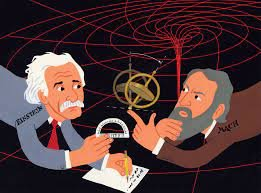Mass is both a property of a physical body and a measure of its resistance to acceleration (a change in its state of motion) when a net force is applied.

Physicists who are proponents of the Standard Model realized in order for it to agree with observations it was necessary to imagine a new field called the Higgs which must exist everywhere in the universe to explain what mass is and its resistance to acceleration. However, shoring up existing theories by inventing new theoretical components to the universe is dangerous, and in the past led physicists to hypothesize a universal aether but the more math they did, the more they realized that the Higgs field simply had to be real. The only problem? By the very way they’d defined it, the Higgs field would be virtually impossible to observe.However, if they had spent the time to analyze the conceptual foundations of Einstein, they would have realized that he had already explained mass and its resistance to motion in terms of his math and observations.

He was able to explained the physicality of mass in terms of an increase in the energy density of space while defining its resistance to a change in motion terms of it occupying a “flat” region in space-time.  This is because he showed us the increase in the energy density caused by mass results in the “surface” of space-time to be curved. Therefore, one can assume a mass moving at a constant velocity MUST be moving through “flat” region of it whose energy level is constant because if it was not, it would be accelerated.

Yet this also allows one to define relative motion in terms of the different energy levels they occupy in space-time.  For example, Einstein’s equation E=mc^2 that defines the

equivalence

between mass and energy tells us the magnitude of them would be directly related to mass.  In other words, a large mass that is not in relative motion with respect to smaller one would occupy a higher energy level.

However, if they were in relative motion one would have to add the energy associated with its motion to determine their relative energy levels.  Putting it another, way the difference between the energy levels of two objects in motion would not only be related to their mass but also to their relative velocities. Therefore, according to Einstein relative motion occurs when the difference between their energy levels in space-time exceeds what is associated with their masses.  Additionally, it tells us to change motion of mass one must also change its energy level.

(The reason all motion is relative is because as was just shown Einstein defined it only in terms of the difference in the energy level between masses in space-time.)

This conclusion is supported by the fact that Einstein derived the force of gravity in terms of a change in the energy levels occupied by a mass as it moves along a curvature in the “surface” of space-time.

This provides an explanation of the resistance, force, or energy required to change the motion of a mass that is consistent with Einstein definition of gravity because as was mentioned earlier the change in motion or acceleration of objects in a gravitational field is a result of them moving through different energy level in space-time. This suggests the resistance or force required to overcome the resistance or a change in motion of a mass is a result of the energy required to change the energy level it is occupying in space time.

This means that one may not have to as some have suggested “invent new theoretical components” to define mass and its resistance a change in its state of motion because as was shown above Einstein equation E=mc^2 defines its physicality in terms of the energy density of space-time while he showed that one can derive its resistance to a change in motion in terms of the force required to change the energy level it occupies in space-time.